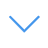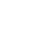# Math Puzzles CG

Math Puzzles CG is one of the Math Games that you can play on UGameZone.com for free. You need to calculate the number of each pattern according to the information provided in the game. Finally, the final answer to the question is obtained. This game can be as addition, subtraction, multiplication and division and requires strong logical thinking. Have fun.

Read Moreاضغط على 【Esc】 للخروج من وضع ملء الشاشة

# Math Puzzles CG

Math Puzzles CG is one of the Math Games that you can play on UGameZone.com for free. You need to calculate the number of each pattern according to the information provided in the game. Finally, the final answer to the question is obtained. This game can be as addition, subtraction, multiplication and division and requires strong logical thinking. Have fun.لتشغيل اللعبة ، تحتاج إلى السماح بتشغيل Adobe Flash Player في متصفحك.

You can use UGameZone Flash Enabler plugin to enable Flash automatically. Or you can follow our instructions to enable Flash manually.

play automatically

## Game Ratings

81%

يلعب 1040

تقييم 81%

الأصوات 776

776
152
Category
Math Games
الكلمات
وصف

Math Puzzles CG is one of the Math Games that you can play on UGameZone.com for free. You need to calculate the number of each pattern according to the information provided in the game. Finally, the final answer to the question is obtained. This game can be as addition, subtraction, multiplication and division and requires strong logical thinking. Have fun.

Step 1
Step 2